## If y=3x+4 and y=2x+8 find the value of both x and y

Question

If y=3x+4 and y=2x+8 find the value of both x and y

in progress 0
5 months 2021-08-27T22:40:00+00:00 1 Answers 2 views 0

x=4, y=16

Step-by-step explanation:

Since y is alone on one side of both equations, that means that the equations are equal.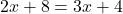Put like terms on the same side of the equations. I put the numbers on the left and the Xs on the right. To do this subtract 4 from both sides. You will get: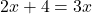Next, I subtracted the 2x from both sides;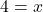For y, just substitute the x in the equation with 4. Will work for either equation.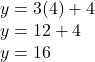Or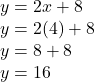Therefore, y=16